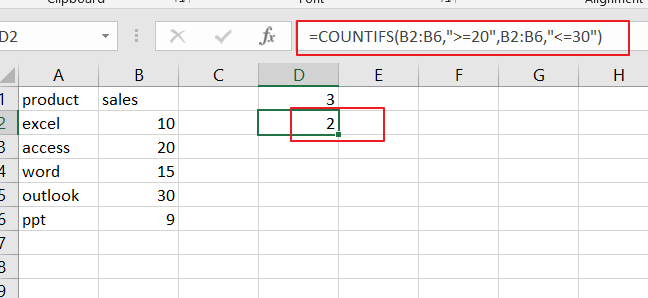# How to Count Number by Range with COUNTIFS Function in Excel

This post will guide you how to Count Number by Range with COUNTIFS function in Excel 2013/2016 or Excel office 365. How do I count the number of values that fall with in a specified range by using the COUNTIFS function in Excel. And you should know that the COUNTIFS function can be used to count the number of cells that meet one or multiple criteria in a given range in Excel. And the post will show you a simple example to count numbers by range with COUNTIFS function.

The Syntax of COUNTIFS function is as below:

=COUNTIFS(range1,criteria1,[range2,criteria2]…)

Where,

Range1 is the data range that will be counted using the criteria1

Criteria1: the condition that determines which cells should be counted

## COUNT NUMBER by Range with COUNTIFS

For Example, you have a data of list in both A2:A6 and B2:B6 and you wish to count the number of sales between the range of 10 to 20, and you can do the following steps:

#1 select Cell D1

#2 enter the following formula based on the COUNTIFS function:

=COUNTIFS(B2:B6,”>=10”,B2:B6,”<=20”)

Then you need to press Enter key to apply this formula. And it will count the cells in range B2:B6 with values between 10 and 20. And the operator “>=” means that “greater than or equal to” and “<=” means that “less than or equal to”.

The formula returns the value 3 in Cell D1. And it means that there are three values between 10 and 20 in your given range B2:B6.

### This formula is the same as in the previous example. And you just only need to change the lowest value is 20, and the highest value is 30. Then this formula will count the cells in range B2:B6 with values between 20 and 30.### Related Functions

• Excel COUNTIFS function
The Excel COUNTIFS function returns the count of cells in a range that meet one or more criteria. The syntax of the COUNTIFS function is as below:= COUNTIFS(criteria_range1, criteria1, [criteria_range2, criteria2]…)…